Next: Ellipsoidal Potential Theory Up: Non-Cartesian Coordinates Previous: Spherical Coordinates

# Exercises

1. Find the Cartesian components of the basis vectors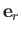,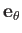, and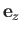of the cylindrical coordinate system. Verify that the vectors are mutually orthogonal. Do the same for the basis vectors,, and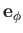of the spherical coordinate system.

2. Use cylindrical coordinates to prove that the volume of a right cylinder of radius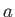and lengthis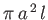. Demonstrate that the moment of inertia of a uniform cylinder of mass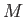and radiusabout its symmetry axis is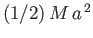.

3. Use spherical coordinates to prove that the volume of a sphere of radiusis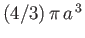. Demonstrate that the moment of inertia of a uniform sphere of massand radiusabout an axis passing through its center is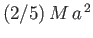.

4. For what value(s) of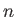is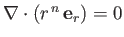, whereis a spherical coordinate?

5. For what value(s) ofis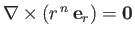, whereis a spherical coordinate?

1. Find a vector field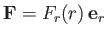satisfying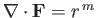for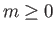. Here,is a spherical coordinate.
2. Use the divergence theorem to show that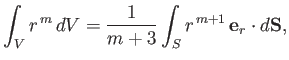whereis a volume enclosed by a surface.
3. Use the previous result (for) to demonstrate that the volume of a right cone is one third the volume of the right cylinder having the same base and height.

6. The electric field generated by a-directed electric dipole of moment, located at the origin, is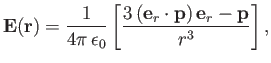where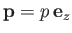, andis a spherical coordinate. Find the components ofin the spherical coordinate system. Calculate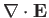and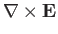.

7. Show that the parabolic cylindrical coordinates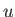,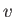,, defined by the equations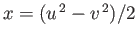,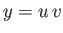,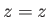, where,,are Cartesian coordinates, are orthogonal. Find the scale factors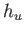,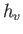,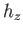. What shapes are the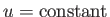and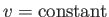surfaces? Write an expression for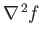in parabolic cylindrical coordinates.

8. Show that the elliptic cylindrical coordinates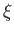,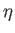,, defined by the equations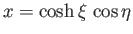,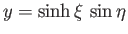,, where,,are Cartesian coordinates, and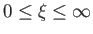,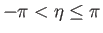, are orthogonal. Find the scale factors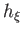,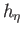,. What shapes are the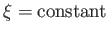and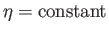surfaces? Write an expression forin elliptical cylindrical coordinates.Next: Ellipsoidal Potential Theory Up: Non-Cartesian Coordinates Previous: Spherical Coordinates
Richard Fitzpatrick 2016-03-31